# Python | Pandas MultiIndex.from_product()

• Last Updated : 24 Dec, 2018

Python is a great language for doing data analysis, primarily because of the fantastic ecosystem of data-centric python packages. Pandas is one of those packages and makes importing and analyzing data much easier.

Pandas` MultiIndex.from_product()` function make a MultiIndex from the cartesian product of multiple iterables.

Syntax: MultiIndex.from_product(iterables, sortorder=None, names=None)

Parameters :
iterables : Each iterable has unique labels for each level of the index.
sortorder : Level of sortedness (must be lexicographically sorted by that level).
names : Names for the levels in the index.

Returns: index : MultiIndex

Example #1: Use `MultiIndex.from_product()` function to construct a MultiIndex from the cartesian product of multiple iterables.

 `# importing pandas as pd``import` `pandas as pd`` ` `# Create the first iterable``Price ``=``[``20``, ``35``, ``60``, ``85``]`` ` `# Create the second iterable``Name ``=``[``'Vanilla'``, ``'Strawberry'``]`` ` `# Print the first iterable``print``(Price)`` ` `# Print the second iterable``print``(``"\n"``, Name)`

Output :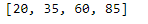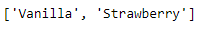Now let’s create the MultiIndex using the above two iterables.

 `# Creating the MultiIndex``midx ``=` `pd.MultiIndex.from_product([Name, Price],``                       ``names ``=``[``'Name'``, ``'Price'``])`` ` `# Print the MultiIndex``print``(midx)`

Output :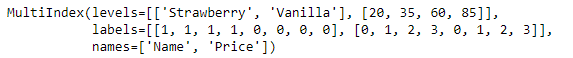As we can see in the output, the function has created a MultiIndex object using the cartesian product of these two iterables.

Example #2: Use `MultiIndex.from_product()` function to construct a MultiIndex from the cartesian product of multiple iterables.

 `# importing pandas as pd``import` `pandas as pd`` ` `# Create the first iterable``Snake ``=``[``'Viper'``, ``'Cobra'``]`` ` `# Create the second iterable``Variety ``=``[``'Brown'``, ``'Yellow'``, ``'Black'``]`` ` `# Print the first iterable``print``(Snake)`` ` `# Print the second iterable``print``(``"\n"``, Variety)`

Output :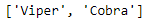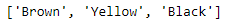Now let’s create the MultiIndex using the above two iterables.

 `# Creating the MultiIndex``midx ``=` `pd.MultiIndex.from_product([Snake, Variety], ``                       ``names ``=``[``'Snake'``, ``'Variety'``])`` ` `# Print the MultiIndex``print``(midx)`

Output :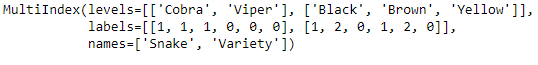The function has created a MultiIndex using the two iterables.

My Personal Notes arrow_drop_up